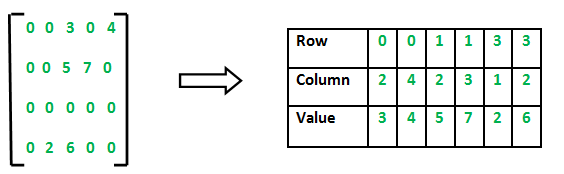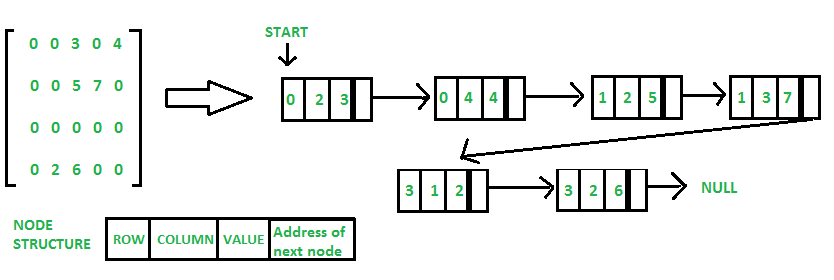Related Articles

# Sparse Matrix and its representations | Set 1 (Using Arrays and Linked Lists)

• Difficulty Level : Medium
• Last Updated : 25 Mar, 2021

A matrix is a two-dimensional data object made of m rows and n columns, therefore having total m x n values. If most of the elements of the matrix have 0 value, then it is called a sparse matrix.

Why to use Sparse Matrix instead of simple matrix ?

• Storage: There are lesser non-zero elements than zeros and thus lesser memory can be used to store only those elements.
• Computing time: Computing time can be saved by logically designing a data structure traversing only non-zero elements..

Example:

```0 0 3 0 4
0 0 5 7 0
0 0 0 0 0
0 2 6 0 0```

Representing a sparse matrix by a 2D array leads to wastage of lots of memory as zeroes in the matrix are of no use in most of the cases. So, instead of storing zeroes with non-zero elements, we only store non-zero elements. This means storing non-zero elements with triples- (Row, Column, value).

Sparse Matrix Representations can be done in many ways following are two common representations:

1. Array representation

Method 1: Using Arrays:
2D array is used to represent a sparse matrix in which there are three rows named as

• Row: Index of row, where non-zero element is located
• Column: Index of column, where non-zero element is located
• Value: Value of the non zero element located at index – (row,column)## C++

 `// C++ program for Sparse Matrix Representation``// using Array``#include` `int` `main()``{``    ``// Assume 4x5 sparse matrix``    ``int` `sparseMatrix =``    ``{``        ``{0 , 0 , 3 , 0 , 4 },``        ``{0 , 0 , 5 , 7 , 0 },``        ``{0 , 0 , 0 , 0 , 0 },``        ``{0 , 2 , 6 , 0 , 0 }``    ``};` `    ``int` `size = 0;``    ``for` `(``int` `i = 0; i < 4; i++)``        ``for` `(``int` `j = 0; j < 5; j++)``            ``if` `(sparseMatrix[i][j] != 0)``                ``size++;` `    ``// number of columns in compactMatrix (size) must be``    ``// equal to number of non - zero elements in``    ``// sparseMatrix``    ``int` `compactMatrix[size];` `    ``// Making of new matrix``    ``int` `k = 0;``    ``for` `(``int` `i = 0; i < 4; i++)``        ``for` `(``int` `j = 0; j < 5; j++)``            ``if` `(sparseMatrix[i][j] != 0)``            ``{``                ``compactMatrix[k] = i;``                ``compactMatrix[k] = j;``                ``compactMatrix[k] = sparseMatrix[i][j];``                ``k++;``            ``}` `    ``for` `(``int` `i=0; i<3; i++)``    ``{``        ``for` `(``int` `j=0; j

## Java

 `// Java program for Sparse Matrix Representation``// using Array``class` `GFG``{` `    ``public` `static` `void` `main(String[] args)``    ``{``        ``int` `sparseMatrix[][]``                ``= {``                    ``{``0``, ``0``, ``3``, ``0``, ``4``},``                    ``{``0``, ``0``, ``5``, ``7``, ``0``},``                    ``{``0``, ``0``, ``0``, ``0``, ``0``},``                    ``{``0``, ``2``, ``6``, ``0``, ``0``}``                ``};` `        ``int` `size = ``0``;``        ``for` `(``int` `i = ``0``; i < ``4``; i++)``        ``{``            ``for` `(``int` `j = ``0``; j < ``5``; j++)``            ``{``                ``if` `(sparseMatrix[i][j] != ``0``)``                ``{``                    ``size++;``                ``}``            ``}``        ``}` `        ``// number of columns in compactMatrix (size) must be``        ``// equal to number of non - zero elements in``        ``// sparseMatrix``        ``int` `compactMatrix[][] = ``new` `int``[``3``][size];` `        ``// Making of new matrix``        ``int` `k = ``0``;``        ``for` `(``int` `i = ``0``; i < ``4``; i++)``        ``{``            ``for` `(``int` `j = ``0``; j < ``5``; j++)``            ``{``                ``if` `(sparseMatrix[i][j] != ``0``)``                ``{``                    ``compactMatrix[``0``][k] = i;``                    ``compactMatrix[``1``][k] = j;``                    ``compactMatrix[``2``][k] = sparseMatrix[i][j];``                    ``k++;``                ``}``            ``}``        ``}` `        ``for` `(``int` `i = ``0``; i < ``3``; i++)``        ``{``            ``for` `(``int` `j = ``0``; j < size; j++)``            ``{``                ``System.out.printf(``"%d "``, compactMatrix[i][j]);``            ``}``            ``System.out.printf(``"\n"``);``        ``}``    ``}``}` `/* This code contributed by PrinciRaj1992 */`

## Python3

 `# Python program for Sparse Matrix Representation``# using arrays` `# assume a sparse matrix of order 4*5``# let assume another matrix compactMatrix``# now store the value,row,column of arr1 in sparse matrix compactMatrix` `sparseMatrix ``=` `[[``0``,``0``,``3``,``0``,``4``],[``0``,``0``,``5``,``7``,``0``],[``0``,``0``,``0``,``0``,``0``],[``0``,``2``,``6``,``0``,``0``]]` `# initialize size as 0``size ``=` `0` `for` `i ``in` `range``(``4``):``    ``for` `j ``in` `range``(``5``):``        ``if` `(sparseMatrix[i][j] !``=` `0``):``            ``size ``+``=` `1` `# number of columns in compactMatrix(size) should``# be equal to number of non-zero elements in sparseMatrix``rows, cols ``=` `(``3``, size)``compactMatrix ``=` `[[``0` `for` `i ``in` `range``(cols)] ``for` `j ``in` `range``(rows)]` `k ``=` `0``for` `i ``in` `range``(``4``):``    ``for` `j ``in` `range``(``5``):``        ``if` `(sparseMatrix[i][j] !``=` `0``):``            ``compactMatrix[``0``][k] ``=` `i``            ``compactMatrix[``1``][k] ``=` `j``            ``compactMatrix[``2``][k] ``=` `sparseMatrix[i][j]``            ``k ``+``=` `1` `for` `i ``in` `compactMatrix:``    ``print``(i)` `# This code is contributed by MRINALWALIA`

Output:

```0 0 1 1 3 3
2 4 2 3 1 2
3 4 5 7 2 6 ```

In linked list, each node has four fields. These four fields are defined as:

• Row: Index of row, where non-zero element is located
• Column: Index of column, where non-zero element is located
• Value: Value of the non zero element located at index – (row,column)
• Next node: Address of the next node## C++

 `// C++ program for sparse matrix representation.``// Using Link list``#include``using` `namespace` `std;` `// Node class to represent link list``class` `Node``{``    ``public``:``    ``int` `row;``    ``int` `col;``    ``int` `data;``    ``Node *next;``};` `// Function to create new node``void` `create_new_node(Node **p, ``int` `row_index,``                     ``int` `col_index, ``int` `x)``{``    ``Node *temp = *p;``    ``Node *r;``    ` `    ``// If link list is empty then``    ``// create first node and assign value.``    ``if` `(temp == NULL)``    ``{``        ``temp = ``new` `Node();``        ``temp->row = row_index;``        ``temp->col = col_index;``        ``temp->data = x;``        ``temp->next = NULL;``        ``*p = temp;``    ``}``    ` `    ``// If link list is already created``    ``// then append newly created node``    ``else``    ``{``        ``while` `(temp->next != NULL)  ``            ``temp = temp->next;``            ` `        ``r = ``new` `Node();``        ``r->row = row_index;``        ``r->col = col_index;``        ``r->data = x;``        ``r->next = NULL;``        ``temp->next = r;``    ``}``}` `// Function prints contents of linked list``// starting from start``void` `printList(Node *start)``{``    ``Node *ptr = start;``    ``cout << ``"row_position:"``;``    ``while` `(ptr != NULL)``    ``{``        ``cout << ptr->row << ``" "``;``        ``ptr = ptr->next;``    ``}``    ``cout << endl;``    ``cout << ``"column_position:"``;` `    ``ptr = start;``    ``while` `(ptr != NULL)``    ``{``        ``cout << ptr->col << ``" "``;``        ``ptr = ptr->next;``    ``}``    ``cout << endl;``    ``cout << ``"Value:"``;``    ``ptr = start;``    ` `    ``while` `(ptr != NULL)``    ``{``        ``cout << ptr->data << ``" "``;``        ``ptr = ptr->next;``    ``}``}` `// Driver Code``int` `main()``{``    ` `    ``// 4x5 sparse matrix``    ``int` `sparseMatrix = { { 0 , 0 , 3 , 0 , 4 },``                               ``{ 0 , 0 , 5 , 7 , 0 },``                               ``{ 0 , 0 , 0 , 0 , 0 },``                               ``{ 0 , 2 , 6 , 0 , 0 } };``    ` `    ``// Creating head/first node of list as NULL``    ``Node *first = NULL;``    ``for``(``int` `i = 0; i < 4; i++)``    ``{``        ``for``(``int` `j = 0; j < 5; j++)``        ``{``            ` `            ``// Pass only those values which``            ``// are non - zero``            ``if` `(sparseMatrix[i][j] != 0)``                ``create_new_node(&first, i, j,``                                ``sparseMatrix[i][j]);``        ``}``    ``}``    ``printList(first);` `    ``return` `0;``}` `// This code is contributed by ronaksuba`

## C

 `// C program for Sparse Matrix Representation``// using Linked Lists``#include``#include` `// Node to represent sparse matrix``struct` `Node``{``    ``int` `value;``    ``int` `row_position;``    ``int` `column_postion;``    ``struct` `Node *next;``};` `// Function to create new node``void` `create_new_node(``struct` `Node** start, ``int` `non_zero_element,``                     ``int` `row_index, ``int` `column_index )``{``    ``struct` `Node *temp, *r;``    ``temp = *start;``    ``if` `(temp == NULL)``    ``{``        ``// Create new node dynamically``        ``temp = (``struct` `Node *) ``malloc` `(``sizeof``(``struct` `Node));``        ``temp->value = non_zero_element;``        ``temp->row_position = row_index;``        ``temp->column_postion = column_index;``        ``temp->next = NULL;``        ``*start = temp;` `    ``}``    ``else``    ``{``        ``while` `(temp->next != NULL)``            ``temp = temp->next;` `        ``// Create new node dynamically``        ``r = (``struct` `Node *) ``malloc` `(``sizeof``(``struct` `Node));``        ``r->value = non_zero_element;``        ``r->row_position = row_index;``        ``r->column_postion = column_index;``        ``r->next = NULL;``        ``temp->next = r;` `    ``}``}` `// This function prints contents of linked list``// starting from start``void` `PrintList(``struct` `Node* start)``{``    ``struct` `Node *temp, *r, *s;``    ``temp = r = s = start;` `    ``printf``(``"row_position: "``);``    ``while``(temp != NULL)``    ``{` `        ``printf``(``"%d "``, temp->row_position);``        ``temp = temp->next;``    ``}``    ``printf``(``"\n"``);` `    ``printf``(``"column_postion: "``);``    ``while``(r != NULL)``    ``{``        ``printf``(``"%d "``, r->column_postion);``        ``r = r->next;``    ``}``    ``printf``(``"\n"``);``    ``printf``(``"Value: "``);``    ``while``(s != NULL)``    ``{``        ``printf``(``"%d "``, s->value);``        ``s = s->next;``    ``}``    ``printf``(``"\n"``);``}`  `// Driver of the program``int` `main()``{``   ``// Assume 4x5 sparse matrix``    ``int` `sparseMatric =``    ``{``        ``{0 , 0 , 3 , 0 , 4 },``        ``{0 , 0 , 5 , 7 , 0 },``        ``{0 , 0 , 0 , 0 , 0 },``        ``{0 , 2 , 6 , 0 , 0 }``    ``};` `    ``/* Start with the empty list */``    ``struct` `Node* start = NULL;` `    ``for` `(``int` `i = 0; i < 4; i++)``        ``for` `(``int` `j = 0; j < 5; j++)` `            ``// Pass only those values which are non - zero``            ``if` `(sparseMatric[i][j] != 0)``                ``create_new_node(&start, sparseMatric[i][j], i, j);` `    ``PrintList(start);` `    ``return` `0;``}`

## Python3

 `# Python Program for Representation of``# Sparse Matrix into Linked List` `# Node Class to represent Linked List Node``class` `Node:` `    ``# Making the slots for storing row,``    ``# column, value, and address``    ``__slots__ ``=` `"row"``, ``"col"``, ``"data"``, ``"next"` `    ``# Constructor to initialize the values``    ``def` `__init__(``self``, row``=``0``, col``=``0``, data``=``0``, ``next``=``None``):` `        ``self``.row ``=` `row``        ``self``.col ``=` `col``        ``self``.data ``=` `data``        ``self``.``next` `=` `next`  `# Class to convert Sparse Matrix``# into Linked List``class` `Sparse:` `    ``# Initialize Class Variables``    ``def` `__init__(``self``):``        ``self``.head ``=` `None``        ``self``.temp ``=` `None``        ``self``.size ``=` `0` `    ``# Function which returns the size``    ``# of the Linked List``    ``def` `__len__(``self``):``        ``return` `self``.size` `    ``# Check the Linked List is``    ``# Empty or not``    ``def` `isempty(``self``):``        ``return` `self``.size ``=``=` `0` `    ``# Responsible function to create``    ``# Linked List from Sparse Matrix``    ``def` `create_new_node(``self``, row, col, data):` `        ``# Creating New Node``        ``newNode ``=` `Node(row, col, data, ``None``)` `        ``# Check whether the List is``        ``# empty or not``        ``if` `self``.isempty():``            ``self``.head ``=` `newNode``        ``else``:``            ``self``.temp.``next` `=` `newNode``        ``self``.temp ``=` `newNode` `        ``# Incrementing the size``        ``self``.size ``+``=` `1` `    ``# Function display the contents of``    ``# Linked List``    ``def` `PrintList(``self``):``        ``temp ``=` `r ``=` `s ``=` `self``.head``        ``print``(``"row_position:"``, end``=``" "``)``        ``while` `temp !``=` `None``:``            ``print``(temp.row, end``=``" "``)``            ``temp ``=` `temp.``next``        ``print``()``        ``print``(``"column_postion:"``, end``=``" "``)``        ``while` `r !``=` `None``:``            ``print``(r.col, end``=``" "``)``            ``r ``=` `r.``next``        ``print``()``        ``print``(``"Value:"``, end``=``" "``)``        ``while` `s !``=` `None``:``            ``print``(s.data, end``=``" "``)``            ``s ``=` `s.``next``        ``print``()` `# Driver Code``if` `__name__ ``=``=` `"__main__"``:` `    ``# Creating Object``    ``s ``=` `Sparse()` `    ``# Assuming 4x5 Sparse Matrix``    ``sparseMatric ``=` `[[``0``, ``0``, ``3``, ``0``, ``4``],``                    ``[``0``, ``0``, ``5``, ``7``, ``0``],``                    ``[``0``, ``0``, ``0``, ``0``, ``0``],``                    ``[``0``, ``2``, ``6``, ``0``, ``0``]]``    ``for` `i ``in` `range``(``4``):``        ``for` `j ``in` `range``(``5``):` `            ``# Creating Linked List by only those``            ``# elements which are non-zero``            ``if` `sparseMatric[i][j] !``=` `0``:``                ``s.create_new_node(i, j, sparseMatric[i][j])` `    ``# Printing the Linked List Representation``    ``# of the sparse matrix``    ``s.PrintList()` `    ``# This code is contributed by Naveen Rathore`

Output:

```row_position: 0 0 1 1 3 3
column_postion: 2 4 2 3 1 2
Value: 3 4 5 7 2 6              ```

Other representations:
As a Dictionary where row and column numbers are used as keys and values are matrix entries. This method saves space but sequential access of items is costly.

As a list of list. The idea is to make a list of rows and every item of list contains values. We can keep list items sorted by column numbers.
Sparse Matrix and its representations | Set 2 (Using List of Lists and Dictionary of keys)

This article is contributed by Akash Gupta.If you like GeeksforGeeks and would like to contribute, you can also write an article using contribute.geeksforgeeks.org or mail your article to contribute@geeksforgeeks.org. See your article appearing on the GeeksforGeeks main page and help other Geeks.